Chemguide: Support for CIE A level Chemistry ``` ``` Learning outcome 24: Electrochemistry 24.2: Standard electrode potentials ``` ``` Learning outcomes 24.2.1, 24.2.2, 24.2.3, 24.2.4 and 24.2.5 These statements deal with standard electrode potentials and their uses. I am dealing with these in one block for reasons I will explain below. Before you go on, you should find and read the statements in your copy of the syllabus. Just skim through these quickly to start with, and then come back to individual statements as you need to. ``` ``` Essential background work This topic can be a total nightmare. There are almost as many different ways of approaching this as there are teachers and writers, and most of these ways manage to make the whole thing completely confusing. CIE's syllabus and the teacher support material offer a sensible approach, but some of their earlier exam questions and mark schemes up to 2010 seemed to take a different approach, and what they wanted sometimes seemed inconsistent with the teacher support material. More recent questions have been more reasonable. So what I would like you to do is to get comfortable with this topic without any reference to what the syllabus is asking point-by-point. Once you are comfortable, we will come back and look at the individual statements. On Chemguide, there is a set of five pages about this. They are intended to be read in order, and by the end of them you should have a clear idea of what is happening, and a simple visual way of using electrode potentials with confidence. The section starts with an introduction to redox equilibria, and you will find a link to the next page at the bottom of each page you finish. There are a few important points before you start: You must take your time over this. I would suggest that you don't try to do more than one page at a time, and that you don't move on to the next page until you are completely confident about the current one. On the first page, about 3/4 of the way down, you will find a section about cell conventions, including a quick way of drawing a cell. Up to now, CIE have never used this method, but you should read about it anyway, because it isn't difficult. You will need to know the convention about the sign of the right-hand electrode. You will also find similar cell diagrams on the third page of the sequence. These are getting a bit complicated, and you don't need them. Just skip over them, but take care not to miss any important bits of surrounding text. ``` ``` When you have finished this, you will have covered almost all of what the syllabus and the teacher support material asks, apart from some very simple calculations, and a small amount of new material in statement 24.2.10. It is now time to go through point-by-point to see how it all fits the syllabus, and to try to tie it into the sort of questions that get asked. Don't go on unless you are sure that you understand what you have read so far. ``` ``` Statement 24.2.1(a) The definition of standard electrode potential is just over 3/4 of the way down the first page in the sequence you have just read. Don't forget to learn the conditions relating to the word "standard" from further up that page. ``` ``` Statement 24.2.2 This is about the standard hydrogen electrode. You will find that on the same page. ``` ``` Statement 24.2.3 You will also find the measurement of the standard electrode potentials of metals in contact with their ions on the same page. Measuring the standard electrode potentials of non-metals and their ions (such as chlorine and chloride ions) is discussed on the third page in the sequence, along with ions of the same element in different oxidation states. ``` ``` Statements 24.2.1(b), 24.2.4 and 24.2.5(a) These statements are about overall cell potentials. The standard cell potential is the emf measured when you connect two half-cells together under standard conditions. There are two different situations where this might arise, and they have to be treated slightly differently. You could be given a diagram of two half-cells and asked to calculate the overall cell potential together with the polarity of the electrodes. You could be given an equation with no diagram, and asked to calculate the cell potential. In either case, you would need to look up standard electrode potentials for the half-reactions. CIE asked for a definition of standard cell potential in a question in November 2017. What they wanted was: the potential difference between two half cells (1 mark) standard conditions of 1 atm, 298 K, with solutions having a concentration of 1 mol dm-3 (1 mark) ``` ``` Finding the cell potential and electrode polarity from a diagram The standard electrode potential is actually a special case of a standard cell potential where one of the half-cells is a hydrogen electrode. You can also imagine a cell made up of two half-cells like this: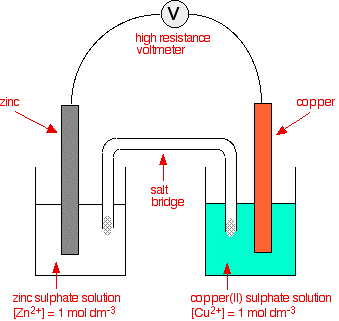The E° values for the two half-cells are: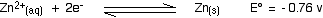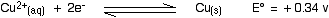The negative sign of the zinc E° value shows that it releases electrons more readily than hydrogen does. The positive sign of the copper E° shows that it releases electrons less readily than hydrogen. So you can work out that in the combined cell in the diagram, the zinc electrode will be relatively more negative, and the copper one relatively more positive. There will be more build up of electrons on the zinc than there are on the copper. Calculating the cell potential in this situation There is a very simple formula for doing this. To find the standard cell potential, you subtract the E° value of the left-hand half-cell from the E° value of the right-hand half-cell. E°cell = E°right - E°left So in this case: E°cell = +0.34 - (-0.76) volts = +1.10 volts Now, remember a convention that you came across early in the series of pages that you have read. The sign of E°cell when calculated in this way represents the sign of the right-hand electrode as you have drawn it. That means that the copper electrode (the right-hand electrode) is the positive one. We have already worked this out of course, but the calculation gives you another check on it. Suppose you had taken the same cell, but drawn it the other way around - with the zinc on the right and the copper on the left. If you had done the same sum, you would get an answer of -1.10 volts. Now that the zinc is the right-hand electrode, the answer shows that this is the negative electrode. The direction of electron flow in the external circuit This is from statement 24.2.5(a), but just follows logically on from what we have been talking about. The two half-cells we have been using as an example are:The position of the zinc equilibrium must be further to the left because it is the more negative. That means that there is a greater build-up of electrons on the zinc at equilibrium than on the copper. If electrons are allowed to flow through the external circuit, then they will obviously flow from where there are more of them to where there are fewer. They will flow from the zinc to the copper. ``` ``` Calculating the cell potential from a given equation If you are given a diagram of a cell, it is really easy to calculate the cell potential. If you are given an equation for an overall reaction, it suddenly gets much more confusing. A CIE question asked you to work out the E° for this reaction (although they made you work out the equation to start with!):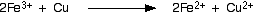The first thing you have to do is to split the equation into two bits so that you can find the electron-half-equations, and the E° values which go with them. In this case, the Fe3+ ions are being converted into Fe2+ ions, and the Cu is being converted into Cu2+ ions. In an exam, you will be given the relevant half-equations and their E° values. You can find this information from the Data section towards the end of the syllabus. Find the two equations you will need, and write them down exactly as they are listed in the table. For the iron equation, you will need Fe3+ ions on one side, and Fe2+ ions on the other. For now, don't worry about which side of the equation they are on. For the copper equation, you will need copper atoms on one side, and Cu2+ ions on the other. Again, don't worry about which side of the equation they are on. The two equations you should have found are: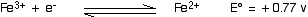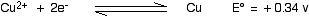Before we look at the calculation, just check that you can see why these values lead to the reaction we are interested in. The iron equilibrium is the more positive, and so will move to the right. The less positive (more negative) copper equilibrium will move to the left. That is exactly what the original equation is saying. Now all you have to do is to take one from the other in order to find the E° of the reaction. But there is obviously a problem here. Which do you take away from which? There isn't a right-hand and left-hand half-cell any more. There is another equation that you can use, and it is probably more likely that you will need this one than the previous one. E°cell = E°red - E°ox Note:  There is a very easy way to remember this equation. The most important thing is the order of the terms on the right-hand side. The "red" comes before the "ox". That is, of course, the same order as in "redox". Let's go back to the original equation again.During this reaction: The Fe3+ ions are being reduced (gain of electrons). The copper is being oxidised (loss of electrons). The E°red in the equation above is the E° value for the half-reaction in which reduction is taking place - in this case, the Fe3+ to Fe2+ change. The value for this is +0.77 v. The E°ox in the equation above is the E° value for the half-reaction in which oxidation is taking place - in this case, the Cu to Cu2+ change. The value for this is +0.34 v. So: E°cell = E°red - E°ox E°cell = +0.77 - (+0.34) = +0.43 v For reasons we will look at in statement 24.2.10, the E° value of a feasible reaction has to be positive. Since you are given the equation which implies that the reaction works, this reaction is obviously feasible, and so the E° value has to be positive. But suppose you weren't given an equation, and don't know whether a reaction is feasible. Suppose you were asked whether iodine would oxidise iron(II) ions to iron(III) ions, and you had to produce a value for the cell potential to confirm your answer. The two E° values for the half-reactions you would need are: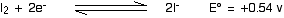Before you do anything else, just look at the numbers and see what answer you would expect. The iron equilibrium has a larger positive E° than the iodine one. The iron one will tend to move to the right and the iodine will be forced to the left. But if you have got iodine and Fe2+ ions, that's where they are already. Any reaction would need them to move the other way. So you wouldn't expect any reaction to occur. Note:  This way of looking at it is covered in a lot more detail on this page from the sequence you read before, and is covered again in statement 24.2.5(b) below. Now let's use the equation. The E°red in the equation above is the E° value for the half-reaction in which reduction is taking place - in this case, that would have to be the I2 to I- change. The value for this is +0.54 v. The E°ox in the equation above is the E° value for the half-reaction in which oxidation is taking place - in this case, the Fe2+ to Fe3+ change. The value for this is +0.77 v. So: E°cell = E°red - E°ox E°cell = +0.54 - (+0.77) = -0.23 v The negative value shows that this reaction isn't possible. To repeat, we will explore why this is in statement 24.2.10 ``` ``` Statement 24.2.5(b) This statement asks you to make predictions about the feasibility of a reaction. You will already have read about this in the set of pages I asked you to look at at the start of this page. It would pay you to read about it again now. You will find it on this page. ``` ``` Go to the Section 24 Menu . . . To return to the list of learning outcomes in Section 24 Go to the CIE Main Menu . . . To return to the list of all the CIE sections Go to Chemguide Main Menu . . . This will take you to the main part of Chemguide. © Jim Clark 2020